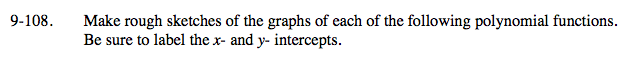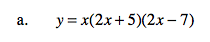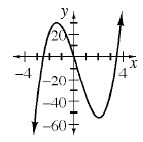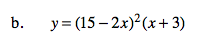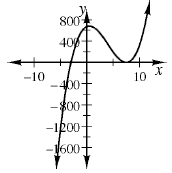Home > A2C > Chapter 9 > Lesson 9.2.3 > Problem9-108

9-108.
1. Make rough sketches of the graphs of each of the following polynomial functions. Be sure to label the x- and y- intercepts. Homework Help ✎

1. y = x(2x + 5)(2x − 7)

2. y = (15 − 2x)2(x + 3)